# GLM: First level analysis#

These are examples focused on showcasing first level models functionality and single subject analysis.

See Analyzing fMRI using GLMs for more details.Example of explicit fixed effects fMRI model fitting

Example of explicit fixed effects fMRI model fitting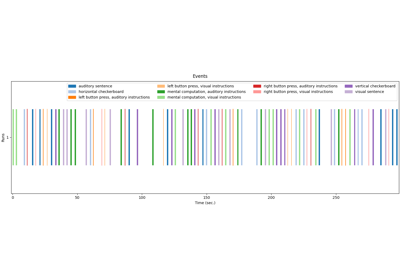Generate an events.tsv file for the NeuroSpin localizer task

Generate an events.tsv file for the NeuroSpin localizer taskDefault Mode Network extraction of ADHD dataset

Default Mode Network extraction of ADHD dataset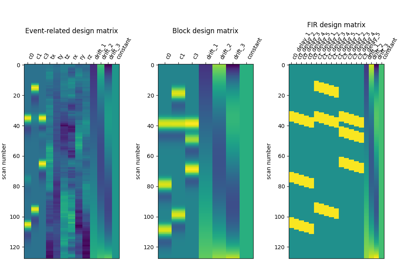Examples of design matrices

Examples of design matrices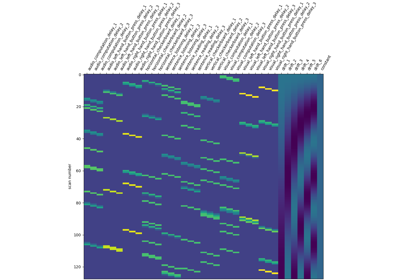Analysis of an fMRI dataset with a Finite Impule Response (FIR) model

Analysis of an fMRI dataset with a Finite Impule Response (FIR) modelSingle-subject data (two sessions) in native space

Single-subject data (two sessions) in native space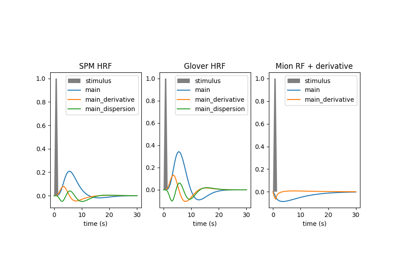Example of MRI response functions

Example of MRI response functionsSimple example of two-session fMRI model fitting

Simple example of two-session fMRI model fittingPredicted time series and residuals

Predicted time series and residuals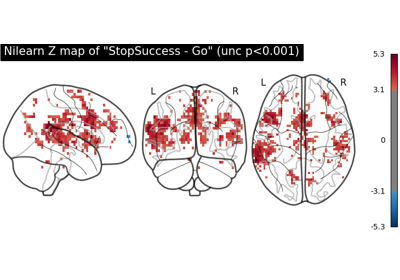First level analysis of a complete BIDS dataset from openneuro

First level analysis of a complete BIDS dataset from openneuroExample of surface-based first-level analysis

Example of surface-based first-level analysisUnderstanding parameters of the first-level model

Understanding parameters of the first-level model